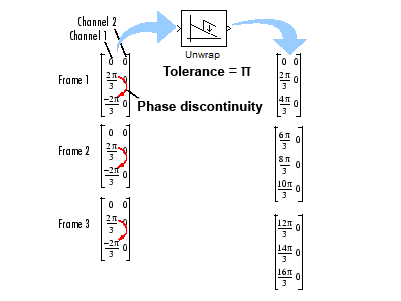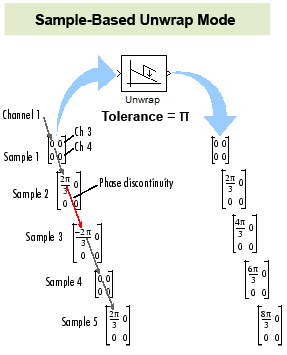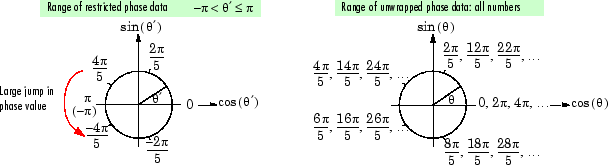Documentation

# Unwrap

Unwrap signal phase

## Library

Signal Operations

`dspsigops`## Description

The Unwrap block unwraps each channel of the input by adding or subtracting appropriate multiples of 2π to each channel element. The input can be a vector or matrix, and must have radian phase entries. The block recognizes phase discontinuities larger than the Tolerance parameter setting. For more information about phase unwrapping, see the Definition of Phase Unwrap.

The block preserves the input size and dimension, and the output port rate equals the input port rate.

### Unwrap Method

The Unwrap block unwraps each channel of its input matrix or input vector by adding 2πk to each successive channel element, and updating k at each phase jump. A phase jump occurs when the difference between two adjacent phase value entries exceeds the value of the Tolerance parameter.

The following code illustrates how the block unwraps the data in a given input channel `u`.

```k=0; % initialize k to 0 i=1; % initialize the counter to 1 alpha=pi; % set alpha to the desired Tolerance. In this case, pi for i = 1:(size(u)-1) yout(i,:)=u(i)+(2*pi*k); % add 2*pi*k to ui if((abs(u(i+1)-u(i)))>(abs(alpha))) %if diff is greater than alpha, increment or decrement k if u(i+1)<u(i) % if the phase jump is negative, increment k k=k+1; else % if the phase jump is positive, decrement k k=k-1; end end end yout((i+1),:)=u(i+1)+(2*pi*k); % add 2*pi*k to the last element of the input```

### Frame-Based Processing

When you configure the block to perform frame-based processing, the block supports two different unwrap modes. In both modes, the block adds 2πk to each input channel's elements, and updates k at each phase discontinuity. The difference between the two modes is how often the block resets the initial phase value (k) to zero. You can choose to unwrap data across frame boundaries (default), or to unwrap only within input frames, by resetting the initial phase value each time a new input frame is received.

#### Unwrapping Across Frame Boundaries

In the default mode, the block ignores boundaries between input frames, and continues to unwrap the data in each channel without resetting the initial phase value to zero. To specify this mode, clear the Do not unwrap phase discontinuities between successive frames check box. The following figure illustrates how the block unwraps data in this mode.#### Unwrapping Within Frames

When you select the Do not unwrap phase discontinuities between successive frames check box, the block treats each frame of input data independently. In this mode, the block resets the initial phase value to zero each time a new input frame is received. The following figure illustrates how the block unwraps data in this mode.### Sample-Based Processing

When you configure the block to perform sample-based processing, the block treats each element of the input as an individual channel. The block unwraps the data in each channel of the input, and does not reset the initial phase to zero each time a new input is received. The following figure illustrates how the block unwraps data when performing sample-based processing.### Definition of Phase Unwrap

Algorithms that compute the phase of a signal often only output phases between –π and π. For instance, such algorithms compute the phase of sin(2π + 3) to be 3, since sin(3) = sin(2π + 3), and since the actual phase, 2π + 3, is not between –π and π. Such algorithms compute the phases of sin(–4π + 3) and sin(16π + 3) to be 3 as well.

Phase unwrap or unwrap is a process often used to reconstruct a signal's original phase. Unwrap algorithms add appropriate multiples of to each phase input to restore original phase values, as illustrated in the following diagram. See Unwrap Method for more information on the unwrap algorithm used by this block.

The following figure illustrates the concept of phase unwrapping.## Parameters

Tolerance

The jump size that the block recognizes as a true phase discontinuity. The default is set to π (rather than a smaller value) to avoid altering legitimate signal features. To increase the block's sensitivity, set the Tolerance to a value slightly less than π.

Input processing

Specify how the block should process the input. You can set this parameter to one of the following options:

• `Columns as channels (frame based)` (default) — When you select this option, the block treats each column of the input as a separate channel.

• `Elements as channels (sample based)` — When you select this option, the block treats each element of the input as a separate channel.

Do not unwrap phase discontinuities between successive frames

When you clear this check box, the block ignores boundaries between input frames and does not reset the initial phase value to zero each time a new input is received. In this mode, the block continuously unwraps the data in each column of the input. When you select this check box, the block treats each frame of input data independently, and resets the initial phase value for each new input frame. See the Frame-Based Processing section for more information.

This parameter is available only when you configure the block to perform frame-based processing. In sample-based processing mode, the block does not reset the initial phase value to zero for each new input. See Sample-Based Processing for more information.

## Supported Data Types

• Double-precision floating point

• Single-precision floating point

 `unwrap` MATLAB